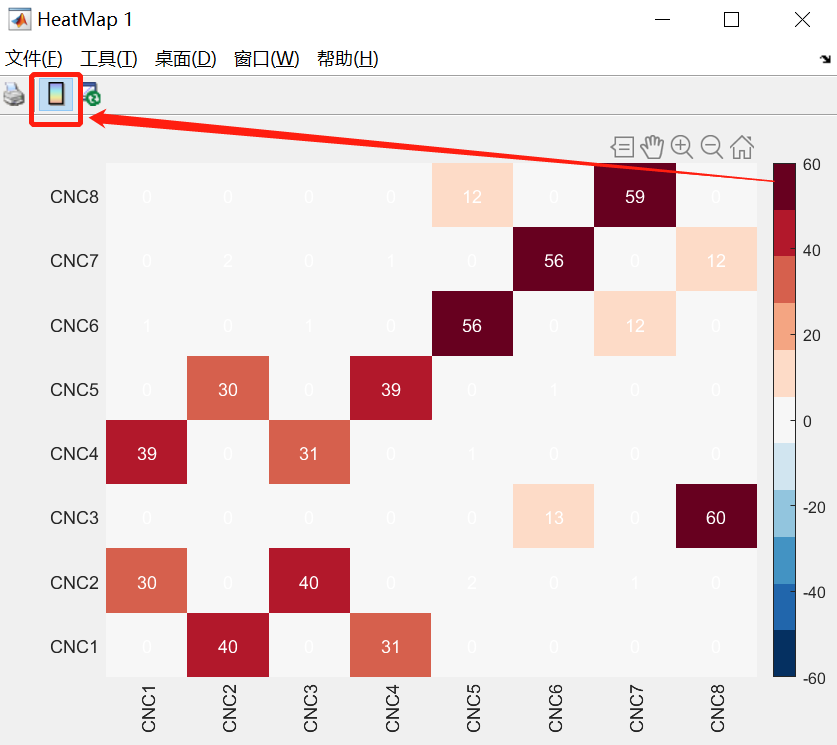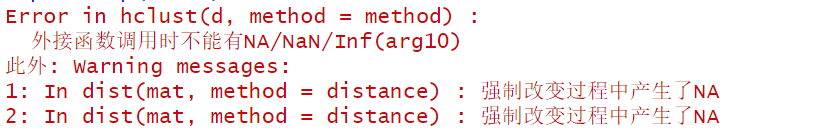• Matlab--使用HeatMap画热图
注意：HeatMap和heatmap是不一样的！
使用HeatMap画热图：
clc;
clear;
data = [0,40,0,31,0,0,0,0;
30,0,40,0,2,0,1,0;
0,0,0,0,0,13,0,60;
39,0,31,0,1,0,0,0;
0,30,0,39,0,1,0,0;
1,0,1,0,56,0,12,0;
0,2,0,1,0,56,0,12;
0,0,0,0,12,0,59,0];
xvalues = {'CNC1','CNC2','CNC3','CNC4','CNC5','CNC6','CNC7','CNC8'};
yvalues = {'CNC1','CNC2','CNC3','CNC4','CNC5','CNC6','CNC7','CNC8'};
yvalues=yvalues';
h=HeatMap(data,'Colormap',redbluecmap,'ColumnLabels',yvalues,'RowLabels',xvalues);
h.Annotate = true;%在热图中显示数据值颜色条可以使用GUI的界面插入colorbar
展开全文matlab
• 使用pheatmap画热图报错 报错内容： Error in hclust(d, method = method) : 外接函数调用时不能有NA/NaN/Inf(arg10) 此外: Warning messages: 1: In dist(mat, method = distance) : 强制改变过程中产生了NA 2: In ...
使用pheatmap画热图报错
报错内容：
Error in hclust(d, method = method) : 外接函数调用时不能有NA/NaN/Inf(arg10) 此外: Warning messages: 1: In dist(mat, method = distance) : 强制改变过程中产生了NA 2: In dist(mat, method = distance) : 强制改变过程中产生了NA
问题详述：
笔者今天先学习了一下R语言和Rstudio的关系，并了解了RStudio是R语言的集成开发环境，一般的任务之间在RStudio中工作就行。本人用Rstudio导入的xls文件。
// 导入excel数据
> heapmap <- read_excel("heapmap.xlsx", sheet = "Sheet3")
//调取pheatmap
> library(pheatmap)
> pheatmap(heapmap)
然后问题就出现了！！！
报错 报错经过一下午的猜测搜索也没找到合适的答案，我猜测是excel的问题，就把随机生成的数据粘贴到新的文本文件，然后导入； 发现问题解决了。
// A code block

主要是row.names=1这个参数必须有，不然会出现报错


总结
这个问题是导入数据的问题，需要row.names=1的这个参数。
解决方案
1：尽量不使用excel导入数据，转换成txt文件再导入； 2：使用参数row.names=1的导入参数
展开全文R语言
• R语言 ggplot 画热图 P值 print(temp) #使用scales包中melt函数将矩阵转换为ggplot需要的data.frame。 #注意：若是所有行名列名都是数字就会转换成matrix 下面展示一些 代码。 // 使用scales包中melt函数将矩阵转换...


R语言 ggplot 画热图 P值

print(temp)#使用reshape2包中melt函数将矩阵转换为ggplot需要的data.frame。 #注意：若是所有行名列名都是数字就会转换成matrix 下面展示一些 代码。
// 使用scales包中melt函数将矩阵转换为ggplot需要的data.frame
library(reshape2)
temp_1<-melt(temp)

// ggplot画图
ggplot(temp_1, aes(as.factor(Var1), Var2)) +
geom_tile(aes(fill = value),colour = "white") +
scale_fill_gradient(name="p-value", low = "red",high = "white",breaks=c(0,0.05,1)) +
theme(axis.text.x = element_text(vjust = 0.5, hjust = 0.5, angle = 90))+
coord_fixed(ratio=1)+
theme(axis.text= element_text(size = 5,family="ARL"))+
theme(plot.margin = unit(c(0.1,0,0,0), unit="mm"))+
labs(x = "position", y = "motif", title = "difference distribution")+ ##调整坐标轴名字
theme(plot.title = element_text(size = 8,hjust = 0.5,family = "ARL" ))+
theme(legend.key.width=unit(3,'mm'),legend.key.height=unit(1,'cm'))+
theme(legend.title = element_text(size = 8)) #调整legend title的字号

做出的图大概是这样，我是将p值大于0.05都调成白色，然后有个简单的排序展开全文ggplot2 r语言
• im = plt.imshow(data, cmap=plt.cm.jet) plt.colorbar(im) plt.show() 最后热图的排序和data排序一致
im = plt.imshow(data, cmap=plt.cm.jet)
plt.colorbar(im)
plt.show()

最后热图的排序和data排序一致
展开全文• 这就是用execl热图绘制步骤如下：    1、 首先把数据填充到execl表格中，在调整表格中每个单元格的宽度和高度让他们都成为一个正方形。 如上图所示，在开始菜单中有行列选线可以调整行高、列宽。   2、下面...数据分析 python
• df = pd.DataFrame(np.random.random((100,5)), columns=["a","b","c","d","e"]) # 计算每对变量之间的相关性 corr_matrix=df.corr() # 半个矩阵 mask = np.zeros_like(corr_matrix) mask[np.triu_indices_from...python seaborn
• 利用插值法，绘画漂亮的热图，可以用于脑电或者近红外光学成像数据绘图
• cellwidth = 15, cellheight = 12, fontsize = 8, filename = "test.pdf") 将热图结果按聚类后的顺序输出 aa=pheatmap(test,scale="row") #热图，归一化，并聚类 image.png # 简要查看热图对象的信息 summary(aa) #...
• 5.画热图 p ggplot(nba.m, aes(variable, Name) ) + geom_tile( aes(fill = rescale) ,colour = "white" ) + scale_fill_gradient(low = "white" ,high = "steelblue" ) p 6.结果展示 ...ggplot2 heatmap R
• 相信很多人都看到过上面这种方块图，有点像“华夫饼图”的升级版，也有点像“热图”的离散版。我在一些临床多组学的文章里面看到过好几次这种图，用它来展示病人的临床信息非常合适，我自己也用R包或者AI过类似的...
• 这里我介绍另外一个方法来绘制，采用现成的软件来，可能图的效果不是很清楚，但至少达到我要求的目的 这个软件叫HeatmapBuider 是由stanford大学用.net开发的一个gui的画图工具 在安装这个软件之前首先得...数据分析 .net 语言 工具 xp
• 让我们用这些统计数据来一个热图，这样就可以大概知道哪个球员哪方面的得分比较高。   热图： ax.imshow(x,cmap=...)   代码如下： import numpy as np import pandas as pd from matplotlib ...
• 聚类热图是我们展示芯片、或者测序结果比较常用的方式，在文章中出现的频率非常高，一般来说，这张图的每行对应一个基因，每列对应一个样本，行和列形成的是基因数*样本数的表格，接下来用不同的颜色代表基因在样本...
• 网上找到的画热图的方法大部分跟我预期都有差距，最后找到了一个代码，然后经过改良可以用来画热图/分布图。 这个代码主要是用到通过hist3这个函数对数据进行分类，然后用scatter函数进行绘制。 以随机生成x和y成...matlab
• ## 画相关性热图

千次阅读 2019-06-12 16:24:00
数据格式如下 Sample CM11 CM12 CM13 CM21 CM22 CM23 CM31 CM32 CM33 CM11 1 0.9627369 0.9884578 0.9841946 0.9762492 0.983613 0.9575127 0.743262 0.6178 CM12 0.9627369 1 0.9616447 0.9405868 0.9354329...
• 热图是很常见的图形展示方式，在◾图形解读系列 | 给你5个示例，你能看懂常用热图使用吗？有详细描述。热图基本原则是用颜色代表数字，让数据呈现更直观、对比更明显。常用来表示不同样品组代表性基......